VK4ADC LC Calculator

24 February 2011

FREEWARE - free to download, free to use, no registration required.

It seems I am often wanting to find out the resonant frequency of an LC circuit and in the past I have utilised an Excel-based spreadsheet. With my current involvement in frequency synthesisers using varicap diodes, I thought it was time to make the task easier and write some dedicated software.

Your interest may not be in VCO's but what tuning range you might expect with (for example) a 4-20pF trimmer capacitor across a 100nH inductor you plan to use to in a two metre transverter front end ( the answer is 107 to 206MHz with 2pF stray C/device input capacity according to this software.... Change that stray/input value to 5pF and it drops to 100 to 167 MHz.).

The software is based on the standard formula for resonant frequency : 1 / (2*Pi*SQRT(L*C)) and allows the user to allow for any circuit stray capacity values (this should include active device input or output capacity values) - this latter "component" being most important when dealing with the high end of the tuning range, more-so than the low end.

Please note that the series and parallel resonance formula is the same so whether you are looking to build a series resonant trap or a standard parallel tuned circuit, the same formula is used and hence this calculator applies.

Just a warning though : the values displayed are to a few decimal places. The accuracy of these decimal places is dependent on things like how long your lead lengths are, how accurately you have estimated stray circuit capacity etc.. I would tend to ignore almost all decimal places in any displayed value (except maybe the calculated inductance) simply because of the physical inaccuracies involved - rather than in the calculation process.

If you wonder how you can find out those minimum and maximum frequencies in practice, attach the parallel LC tuned circuit  to a 2-terminal RF oscillator/device & use a frequency counter ( a good technique ) or measure them with a "dip-meter" { fraught with calibration inaccuracies}.

The calculation does not take into account the Q of the inductor. It is always assumed to be high through it's method of construction. Low-Q inductors are hot generally used in LC parallel circuits anyway.....

The software I wrote doesn't look overly pretty - but it is functional :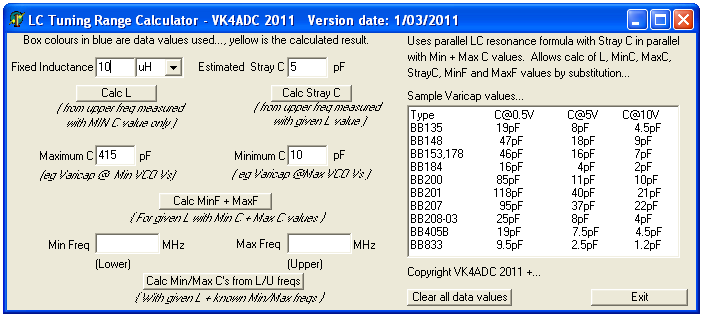Mouse-over to see larger image

To explain the boxes that the user can fill with details :

Fixed Inductance : This is a value of a known inductance, either measured or by an external marking. The standard is in microhenries (uH) although the calculator works with nanoHenry (nH) or millHenry (mH)  values when selected from the dropdown box (versions 1/3/11 and after). If a measurement of upper frequency and minimum capacity of an LC circuit is known, the inductance (L) value can be calculated.

Estimated Stray C : The physical layout of an electronic circuit will contribute some stray capacity across a resonant circuit. This has the effect that it lowers the upper frequency limit that an LC tuned circuit with a variable capacitor can tune to. Rather than ignore it, the user can substitute a nominal value of (for example) 10pF. This value is across both minimum and maximum values of capacitor (CMin & CMax) when involved in the resonace calculations. If a known iductance is used and the upper frequency is measured with a known (minimum) capacitance, the stray circuit capacitance can be calculated.

Maximum C : This is the maximum capacitance value of a physical tuning capacitor ( eg 415pF in a 10-415, 250pF in a 10-250) or a varicap diode (eg 85pF for a BB200 as listed in the data box). The MaxC value can be calculated for a known L and measured upper and lower frequency limits.

Minimum C : This is the minimum capacitance value of a physical tuning capacitor ( eg 10pF in a 10-415, 10pF in a 10-250) or a varicap diode (eg 11pF for a BB200 as listed in the data box for a synth running off a 5V supply). The MinC value can be calculated for a known L and measured upper and lower frequency limits.

Min Freq : This is the minimum or lower end of the frequency range that the given value of inductance (L) will tune to at the maximimum value of tuning capacity ( Maximum C) with the Stray C value in parallel. If an actual value is measured, it can be entered into this box to calculate the L or Maximum C values depending on which actual values are unknown.

Max Freq : This is the maximum or upper end of the frequency range that the given value of inductance (L) will tune to at the minimimum value of tuning capacity ( Minimum C) with the Stray C value in parallel. If an actual value is measured, it can be entered into this box to calculate the L, Minimum C or Stray C values depending on which actual values are unknown.

The following images depict which values are utilised in the available calculation modes. The values backgrounded in light blue are input (or user supplied ) values. The value/s in yellow are the output calculation. The button pushed to achieve the outcome is obvious by the "pushed-button" style inset on the image. Note that the actual software sets the blue text background colours for all fields required for that specific calculation/function, with the yellow outcomes highlighted to make it easier to see which box ( or boxes) contain the results..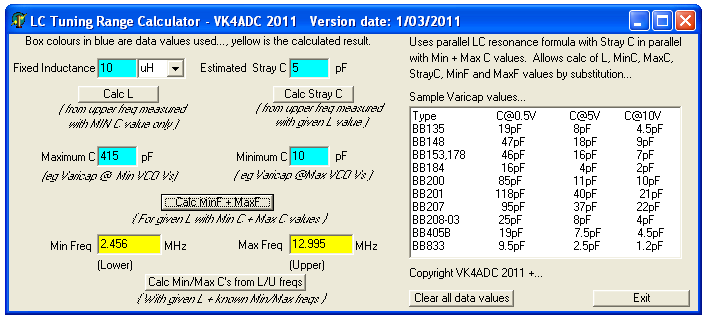Calculating Lower and Upper frequency limits with known inductance, estimated stray capacitance, known maximum and minimum main tuning capacitance values. This example shows a 10uH inductor, a 10-415pF variable capacitor and an estimated 10pF stray circuit capacity.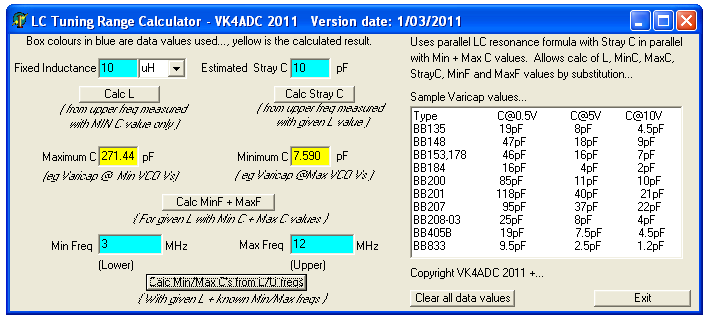Calculating maximum and Minimum capacitance  values with known Lower and Upper frequency limits with known inductance and estimated stray capacitance values. This example shows that we know the inductor value is 10uH and the tuning range is 3 to 12 MHz, again 10pF stray capacity. The variable capacitor is probably a 10 to 250pF with the frequency accuracies given.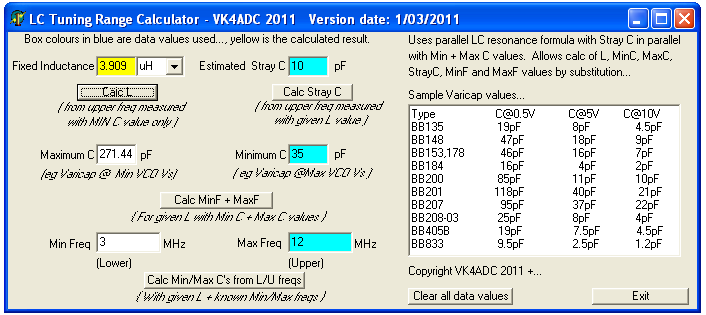Calculating Inductance with a known Upper frequency value and a minimum main tuning capacitance. Estimated stray capacitance affects the accuracy of the calculated inductance value. This shows that an inductor value of 3.9uH is required to resonate with 45pF (35pF min + 10pF stray) at 12 MHz.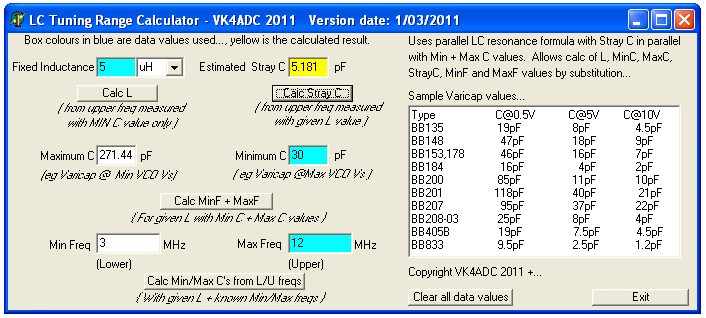Calculating Stray Circuit Capacity Inductance with a known Upper frequency value, a minimum main tuning capacitance and known inductance. If we accurately know the inductor and minimum capacitor values and have measured the upper frequency then we can calculate the actual stray capacity...

From Version.1, an additional button "Clear all data values" has been added toward bottom right that allows the user to clear all data boxes at once so that a completely new set of values can be used.

From Version.2, a  drop-down units box added for the Inductance component. Standard unit is uH with nH and mH as alternatives. see image below.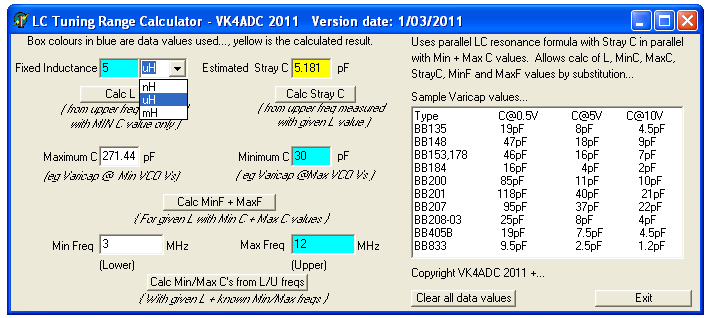Be aware that if you do not place values in all of the compulsory boxes then you will be greeted with an error message that tells you that you need to add more details such that all boxes highlighted in Aqua (/light blue) have valid details in them.

Those are the only calculation modes provided.

You can use it to determine the LC parallel resonant frequency of components you have. Lets say you have a 10-415pF tuning capacitor and a 10uH inductor (it is marked on the actual inductor). If you set Stray C to 0pF (zero), the frequency range will be 2.47 to 15.91 MHz. Allowing for 5pF of Stray C ( a reasonable enough value for some physical layouts), those limits change to 2.45 and 12.99 MHz - so just 5pF of unaccounted circuit capacity changes the upper limit by almost 4 MHz !!!!

Maybe you want to try too pull it down to 1.8 MHz - can you do it ??? Yes, if you add 470pF across the tuning capacitor but that reduces the tuning range to 1.7 to 2.3 MHz. How did I find that out - I simply tried different standard C values in the Stray C box while leaving the L and MaxC and MinC values alone until such time as I had a MinF below 1.8 MHz.

Where is this software ? Right here :           ZIP file  { 167Kb }

No installation process is necessary, just unzip the single LCFreqs.exe file into a folder or the Windows desktop and execute it. No extra files are required or created.
Copyright is claimed. Don't spread the file onto other web sites, just link back to this web page.

The software should run on any 32 or 64 bit Windows and all functionality is within a single EXE file. It was written in Delphi 5 ( a windows-based derivative of Pascal ), a relatively old version these days... but the latest version I have.

Note that the software may be updated if/when any bugs are found in it but the latest version will always be available through the link above. It has already been updated once since originally published on the site.

Software Revisions :  ( apparent through the date at the top of the application)

Initial release : 24/2/2011 ( no file date )

Rev.1 : 26/2/2011 - Clear data button plus text box blue highlighting added.

Rev.2 : 1/3/2011 - Inductance drop-down selector added for nH, uH and mH units.

IF YOU FIND IT USEFUL, OR FIND BUGS IN IT, USE THE "CONTACT ME" FROM THE MENU AT LEFT.